TrigonometryShowing results 31 to 36 of 36, on page 4 of 4 Arbitrary Triangle Trigonometric Definitions Trigonometric Identities Trigonometric Properties
 DESCRIPTION EQUATION Sum and Difference of Angles Identity - Sine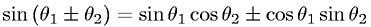Sum and Difference of Angles Identity - Tangent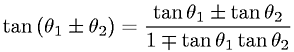Superposition (Addition and Subtraction) of Sine of Angles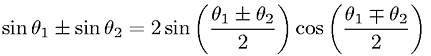Superposition (Addition) of Cosine of Angles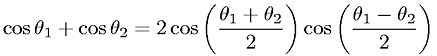Superposition (Subtraction) of Cosine of Angles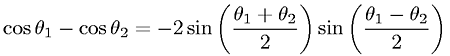Tangent Definition for a Right TriangleGo to page: 1  2  3  4